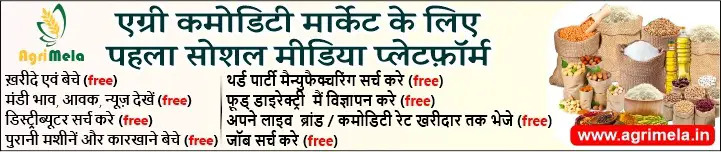Rate this

Rate this

## B.R.K. Canvassing Agent

Rate this

Food Grain Broker, Pulses Broker, Rice Broker in Adoni, Kurnool, Andhra Pradesh, India

Rate this

Rate this

Rate this

Rate this

Rate this

Rate this

Rate this

Rate this

Rate this

Rate this

Rate this

Rate this

Rate this

Rate this

Rate this

Rate this

Rate this

Rate this

Rate this

Rate this

Rate this

Rate this